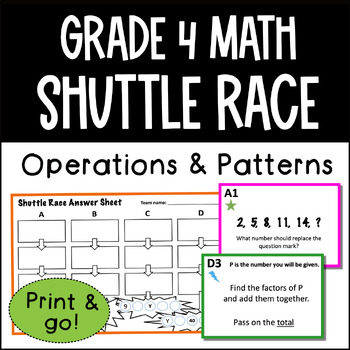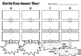# GRADE 4 Shuttle Relay Race OA - Operations & Algebraic ThinkingSubject
Resource Type
File Type
Zip (3 MB|7 pages)
Standards
\$1.50
• Product Description
• Standards
A fun activity covering all the Grade 4 Common Core Operations & Algebraic Thinking standards.

Outline of activity:
Students work in teams of 4, competing to be the first to win the 4 rounds of the relay race.

In their teams of 4 students pair up into twos. The pair with A1 has to start by solving the problem on their card. When the star pair have an answer for A1 they write it on their Answer sheet and pass it to the other pair - this is the only form of
communication allowed between the pairs in the team.

The pair with A2 now have to use this answer to solve A2 - when they get this answer they write it on the sheet and pass it back to the other pair to help them solve
A3 and so on.

When the pair with A4 have put the final answer on the sheet, they then
have to go to you to check their answers.

A great way to review all the key points of the OA topic, as well as encouraging team work and engaging students.

File contains:
- 4 sets of 4 relay questions & Answer sheet
- Instruction sheet

Generate a number or shape pattern that follows a given rule. Identify apparent features of the pattern that were not explicit in the rule itself. For example, given the rule “Add 3” and the starting number 1, generate terms in the resulting sequence and observe that the terms appear to alternate between odd and even numbers. Explain informally why the numbers will continue to alternate in this way.
Find all factor pairs for a whole number in the range 1-100. Recognize that a whole number is a multiple of each of its factors. Determine whether a given whole number in the range 1-100 is a multiple of a given one-digit number. Determine whether a given whole number in the range 1-100 is prime or composite.
Solve multistep word problems posed with whole numbers and having whole-number answers using the four operations, including problems in which remainders must be interpreted. Represent these problems using equations with a letter standing for the unknown quantity. Assess the reasonableness of answers using mental computation and estimation strategies including rounding.
Multiply or divide to solve word problems involving multiplicative comparison, e.g., by using drawings and equations with a symbol for the unknown number to represent the problem, distinguishing multiplicative comparison from additive comparison.
Interpret a multiplication equation as a comparison, e.g., interpret 35 = 5 × 7 as a statement that 35 is 5 times as many as 7 and 7 times as many as 5. Represent verbal statements of multiplicative comparisons as multiplication equations.
Total Pages
7 pages